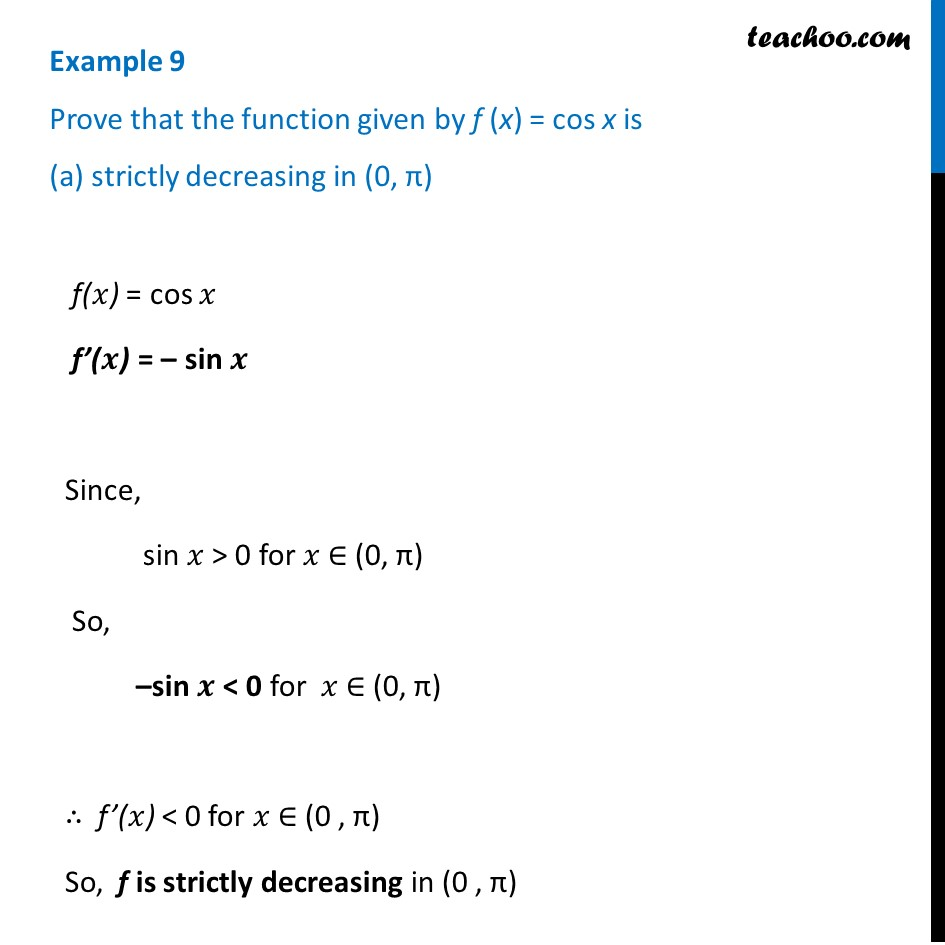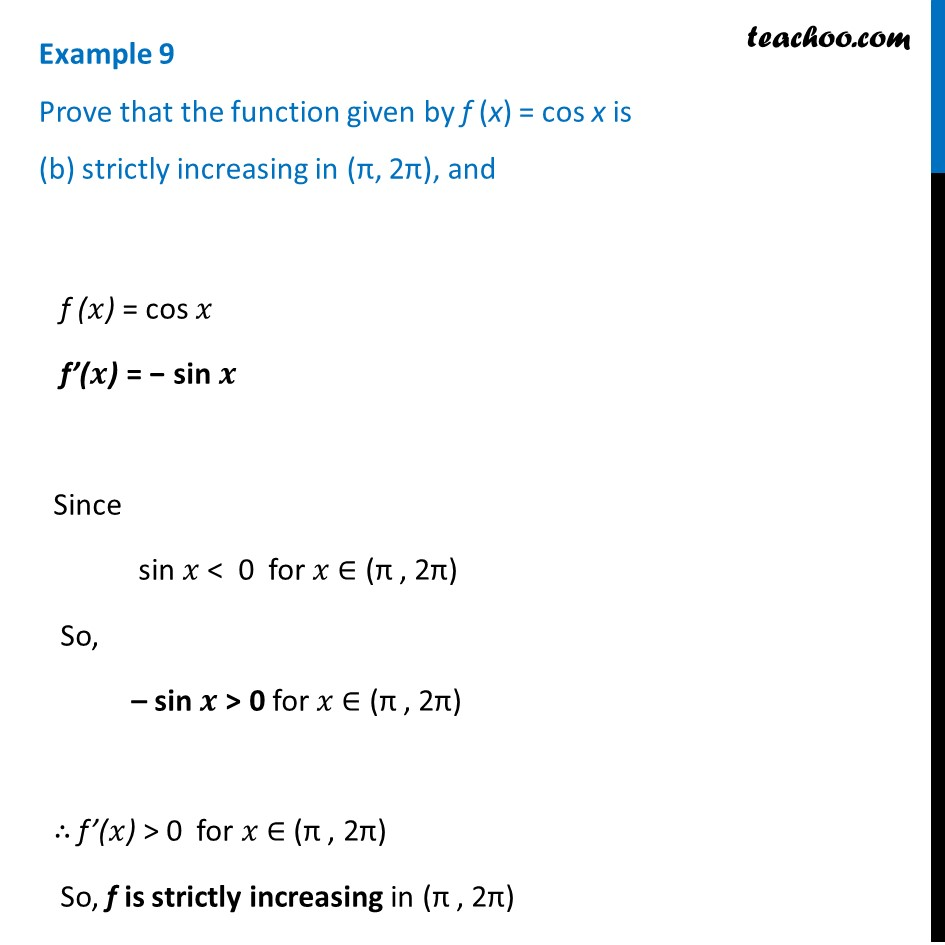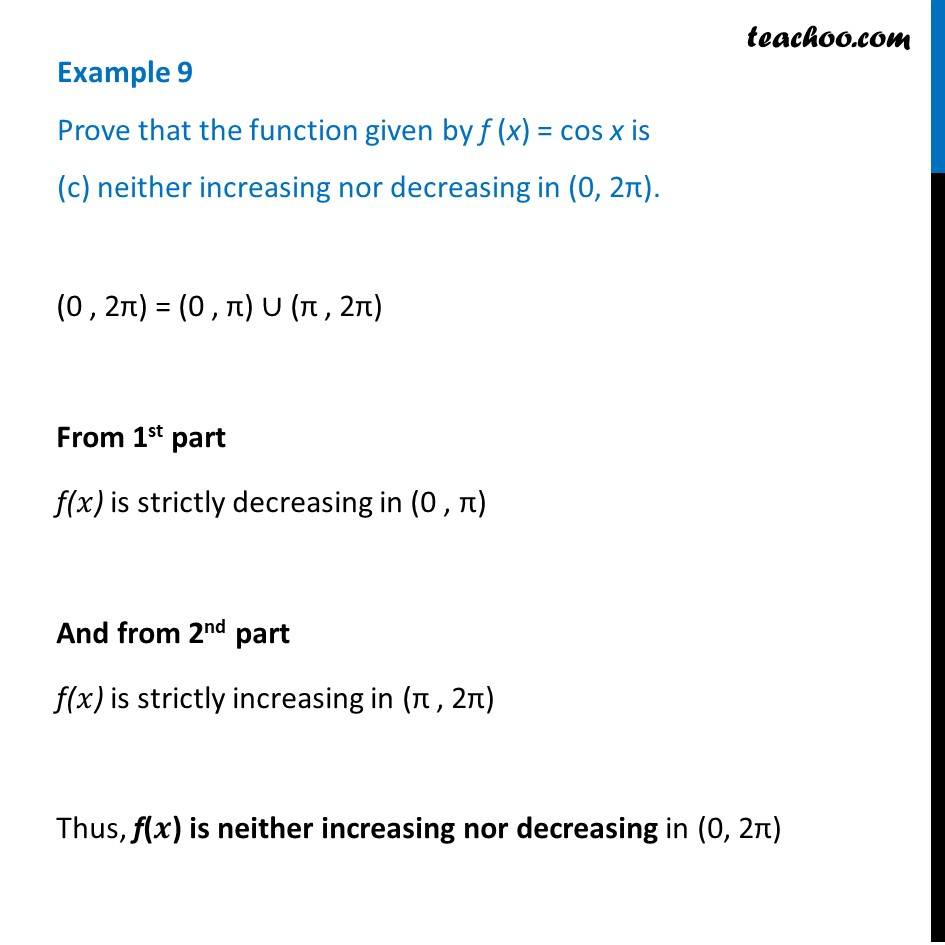Examples

Chapter 6 Class 12 Application of Derivatives
Serial order wiseLearn in your speed, with individual attention - Teachoo Maths 1-on-1 Class

### Transcript

Example 9 Prove that the function given by f (x) = cos x is (a) strictly decreasing in (0, π) f(𝑥) = cos 𝑥 f’(𝒙) = – sin 𝒙 Since, sin 𝑥 > 0 for 𝑥 ∈ (0, π) So, –sin 𝒙 < 0 for 𝑥 ∈ (0, π) ∴ f’(𝑥) < 0 for 𝑥 ∈ (0 , π) So, f is strictly decreasing in (0 , π) Example 9 Prove that the function given by f (x) = cos x is (b) strictly increasing in (π, 2π), and f (𝑥) = cos 𝑥 f’(𝒙) = − sin 𝒙 Since sin 𝑥 < 0 for 𝑥 ∈ (π , 2π) So, – sin 𝒙 > 0 for 𝑥 ∈ (π , 2π) ∴ f’(𝑥) > 0 for 𝑥 ∈ (π , 2π) So, f is strictly increasing in (π , 2π) Example 9 Prove that the function given by f (x) = cos x is (c) neither increasing nor decreasing in (0, 2π).(0 , 2π) = (0 , π) ∪ (π , 2π) From 1st part f(𝑥) is strictly decreasing in (0 , π) And from 2nd part f(𝑥) is strictly increasing in (π , 2π) Thus, f(𝒙) is neither increasing nor decreasing in (0, 2π)